# How to Solve for the Brachistochrone Curve Between Points

October 20, 2015

The shortest route between two points isn’t necessarily a straight line. If by shortest route, we mean the route that takes the least amount of time to travel from point A to point B, and the two points are at different elevations, then due to gravity, the shortest route is the brachistochrone curve. In this blog post, we demonstrate how to use built-in mathematical expressions and the Optimization Module in COMSOL Multiphysics to solve for the brachistochrone curve.

### Finding the Brachistochrone Curve

Imagine letting a marble roll down a curved ramp, such as those seen in roller skate parks, and measuring the time for the marble to roll from point A to point B. Our goal is to redesign the shape of the ramp between the two points, such that it takes the shortest possible time for the marble to travel between them. For simplicity, we consider the ideal situation where friction is neglected and the marble is infinitely small (a point mass).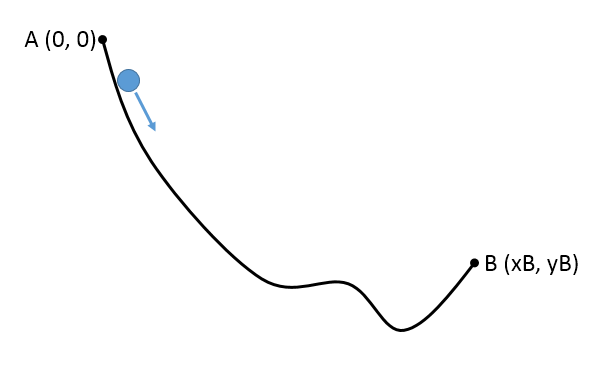The brachistochrone curve is an idealized curve that provides the fastest descent possible. There is actually an analytical solution to this case or, with some derivation work, we can use the PDE functionality of COMSOL Multiphysics to solve the problem. However, since I am a “firm believer of the principle of least action”, a fancy way of saying “a lazy physicist”, we will use the Optimization Module instead.

### Setting Up the COMSOL Multiphysics Model

Assuming point A of our problem is located at the origin (x, y) = (0, 0), the analytical solution for the brachistochrone is a parametric curve of this form:

(1)

\begin{align*}
x(t) &=& r (t-sin(t))\\
y(t) &=& r (-1+cos(t))
\end{align*}

where the parameter r is a constant and the parameter t is the running parameter for the parametric curve and varies linearly from tA to tB along the curve. Typically, when we solve this problem, we are given the location of point B and solve for r and t.

Here, we will start with the analytic solution for the brachistochrone and a known set of r and t that give us the location of point B. We will show how to approximate this analytic solution using the optimization functionality within COMSOL Multiphysics and the Optimization Module.

We will start with a blank model. In fact, we will not add any “component” in the Model Builder; no geometry, physics, or mesh will be needed. This is an interesting example of “a model without a model”.

First, we define a set of global parameters. We set the constant parameter r to 1 and the value of tB for point B to 1.2 \pi. (Keep in mind that A is the origin, so implicitly tA=0.) The location of point B (xB, yB) can then be calculated using Eq. (1), as shown in the screenshot below.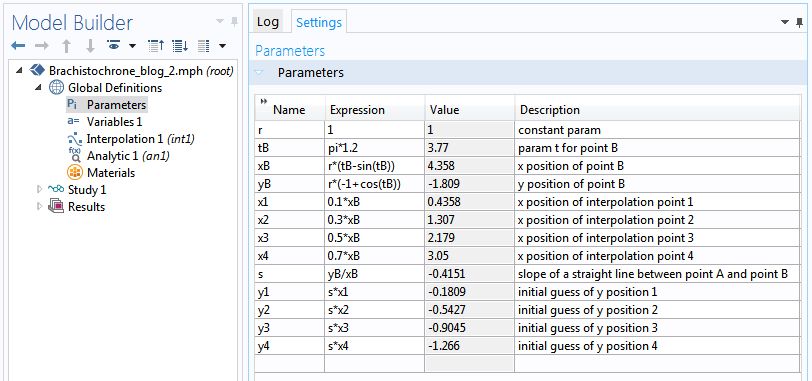Next, we use an interpolation function to approximate the brachistochrone curve. We define the x-positions of a few interpolation points as x1 ~ x4 and give the y-positions ( y1 ~ y4) an initial guess, such that the interpolation points start out on a straight line between point A and point B.

This interpolation function can be set up as shown in following screenshot.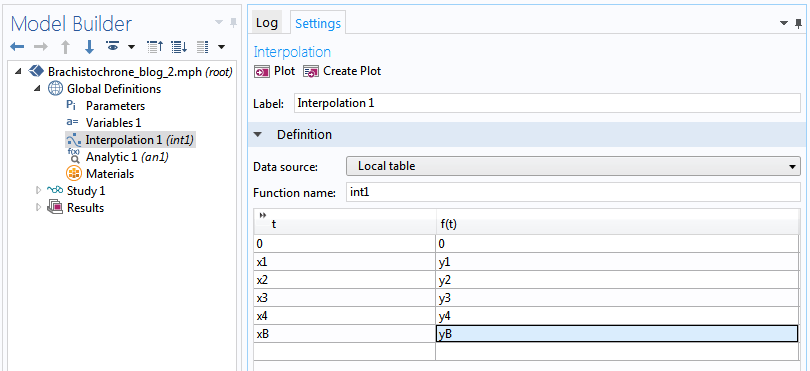You can click the Create Plot button to generate a plot of the interpolation function. For clarity, remove the two extrapolation plots. Next, add a line graph of the analytical solution to the same plot group to compare with the numerical solution later.

To create a plot of the parametric curve, first create a dummy analytic function under the Global Definitions node, setting the upper limit of the argument to tB (under the Plot Parameters section). The sole purpose of this analytic function is to provide a list of t values between 0 and tB for us to draw the parametric curve. Click Create Plot and drag the resulting Line Graph 1 out of 1D Plot Group 2 and into 1D Plot Group 1 (its name will change to Line Graph 2 automatically). For the y-axis data, enter the expression corresponding to Eq. (1): r*(-1+cos(root.x)). Similarly, for the x-axis data, enter  r*(root.x-sin(root.x)).

Note that the COMSOL Multiphysics variable root.x here corresponds to the t parameter in Eq. (1).

Click the Plot button. We now have a graph of the analytic solution (green curve) and the initial guess (blue curve and black dots) as shown below.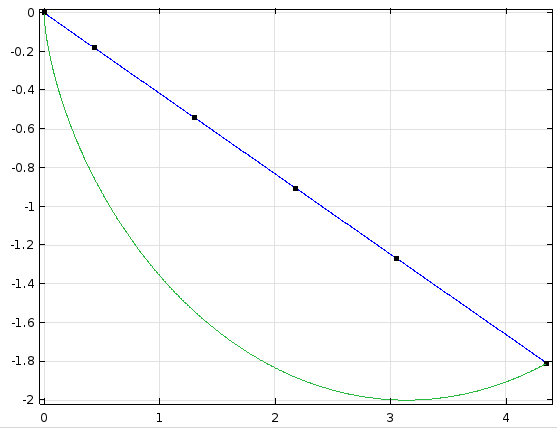### Calculating Travel Time

To calculate the travel time for the marble to roll from point A to point B, we use the assumption that the motion is frictionless, so that all loss in potential energy turns into kinetic energy, which is proportional to the square of the speed. Thus, if the curve is represented by the formula y = f(x), then the instantaneous speed is proportional to the square root of the loss in height: v \propto \sqrt{0-f(x)} (recall that we assume the original height at point A is 0). For an infinitesimal movement of dx in the x-direction, the path length that the marble travels along the curve is ds = dx \sqrt{1+f'(x)^2}. The time it takes to travel this length is simply the length divided by the speed d\tau = ds/v. Therefore, we arrive at an expression for the total travel time for any given curve y = f(x):

T = \int{d\tau}=\int{ds/v}\propto\int_0^{xB}{\sqrt{{1+f'(x)^2}\over{0-f(x)}}dx}

All we have to do now is let the COMSOL software find the curve that minimizes this expression for the travel time.

You may have noticed that we use the “proportional to” symbol (\propto) in the expression for the travel time, since we have neglected to include the mass of the marble and the gravitational acceleration constant in the formula. Since these numbers combine to merely scale the travel time by a constant factor, they do not affect what the curve looks like for the minimization problem. In other words, the brachistochrone curve is independent of the weight of the marble.

Since we use the interpolation function int1 to approximate the curve f(x), we can define a global variable T for the travel time using the formula given above: integrate(sqrt((1+(d(int1(x),x))^2)/max(0-int1(x),eps)),x,0,xB). The extra expression  max(... ,eps) in the denominator prevents divide-by-zero situations, as shown below.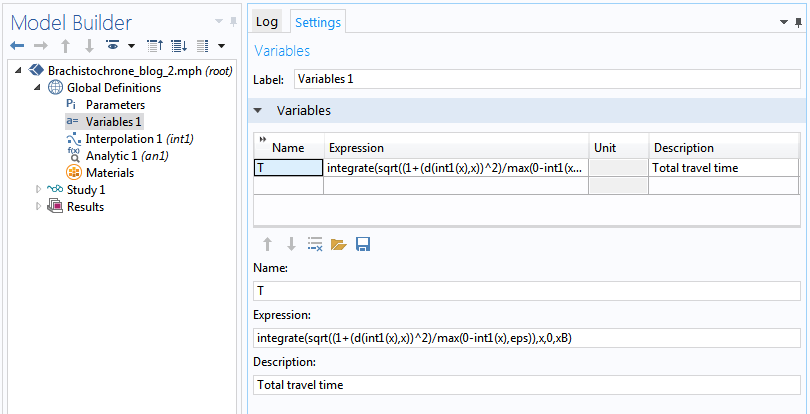### Optimization Solver

Now we are ready to ask the software to minimize the travel time for us. Create an empty study and then right-click to add an Optimization node under it. We can use either the Coordinate Search, Nelder-Mead, BOBYQA, or COBYLA optimization solvers for this “model-free” optimization problem. COBYLA turns out to be the fastest.

In the settings window, under the section Objective Function, enter T for the expression, which by default is minimized. Then, under the section Control Variables and Parameters, use the Add button (a “plus” sign icon) to add the parameters  y1 - y4. The initial value fields are automatically filled with the corresponding values in the global parameters table. Under the Output While Solving section, check the Plot check box. These settings are shown in the screenshot below.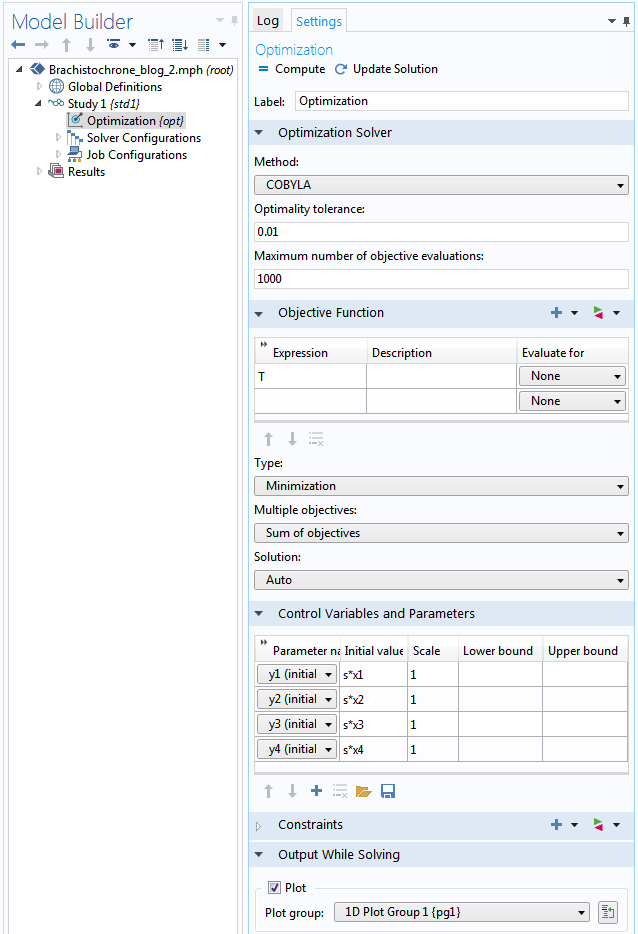Click Compute to watch as the optimization solver moves the interpolation curve up and down until the minimal travel time is reached. Even with the very crude linear interpolation curve using only four interpolation points, the results in the graph below show remarkable agreement with the analytical solution. Higher-order interpolation and more interpolation points will further improve the solution.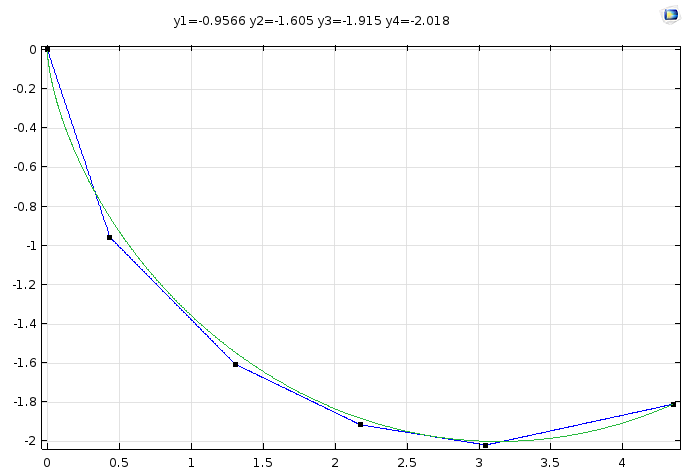### Conclusion

A few years ago, my mentor William Vetterling from ZINK Imaging chatted with a few of us at lunch about how we can use COMSOL Multiphysics to solve anything, since it provides the mathematical tools to handle all kinds of equations encountered in science and technology. This idea eventually evolved into his keynote presentation on the “Library of Babel” at the COMSOL Conference 2012 Boston. We have used an example of a “model without a model” to solve the brachistochrone curve problem by taking advantage of the software’s versatile built-in mathematical expressions and optimization functionality. I hope this demonstration will stimulate more creative usage of COMSOL Multiphysics to tackle more technical challenges.

#### Comments (3)

##### Leave a Comment
Log In | Registration
Loading...##### James Freels
October 21, 2015

I saw this problem for the first time when taking a graduate-level course in “Variational Calculus” or sometimes called “Calculus of Variations” (COV) , whereby one can solve this problem exactly. What I remember about COV was that very few problems can actually be solved this way, but it does offer a mathematical basis from which to proceed to approximate solutions. Indeed, it can be claimed that the Garlerkin Weak formulation, which forms the basis of the finite-element method which COMSOL uses, can be thought of as within the framework of the COV. So, it is very interesting that you have also used COMSOL optimization module to solve the Brachistochrone problem by a discrete method. Once again, this demonstrates the real power that is available within COMSOL. By the way, the instructor for this COV course was a professor of Mechanical Engineering, and he also taught natural convection heat transfer.##### Chien Liu
October 22, 2015

Dear James,
Yes the goal of this post is to demonstrate that COMSOL provides the tools to “solve anything” and the Optimization Module adds more arsenal to the toolbox available to the users. Thank you for sharing your personal perspective on this. We really appreciate it!
Sincerely,
Chien##### said EL-JALLAL
April 26, 2016

Hi Chien Liu, Hi every one,

I try to calculate a complex k photonic band diagram using the weak form (see the paper bellow) . I arrive to define de weak form and all parameters, but my problem is how to define a floquet boundary condition for my periodic function h. The results what I get is wrong and I don’t know where is wrong thing.
paper: https://www.osapublishing.org/oe/abstract.cfm?uri=oe-19-20-19027

For the periodic condition this is what I did:

*In definition—>linear extrusion1: I put the name (linext1) and I choose one face (perpondicular to x) and all source and destination vertises.

*I repeat the same —>linear extrusion2: linext2. and I choose one face (perpondicular to y) and all source and destination vertises.

*I repeat the same —>linear extrusion3: linext3. and I choose one face (perpondicular to z) and all source and destination vertises.

Begin,
*In definition—>variables: select first face, I define: extrcpl_source_pconstr1x=hx, extrcpl_source_pconstr1y=hy, extrcpl_source_pconstr1z=hz,

*I go to another variables which is now the destination: definition—>variable: select the opposit face to the first face I define: pconstr1x=linext1(extrcpl_source_pconstr1x).

I repeat the same for the two other directions (Two variables one first face withextrcpl_source_pconstr1x=hx… and the other one pconstr1x=linext1(extrcpl_source_pconstr1x)…).

End.

Is this right to define the floquet boundary condition of my periodic function h.

If some have an idea I will be appreciate it.
Thank you a lot.
Cordially.
Said.

EXPLORE COMSOL BLOG